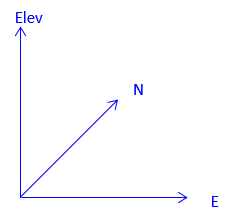### 1. Coordinate System

A coordinate system is a datum for expressing point positions. The system consists of two or three reference axes perpendicular to each other.

In a two dimensional system, one axis is oriented in the direction of a meridian defining North, the remaining axis being East, Figure A-1.Figure A-1Two Dimensional System

In a three dimensional system, the third axis is oriented vertically, in the direction of elevation, Figure X-2.Figure A-2 Three Dimensional System

This section will deal primarily with two dimensional systems although the math involved can be easily expanded into the third dimension.